# Moses::BackwardLanguageModel< Model > Class Template Reference

`#include <Backward.h>`

Inheritance diagram for Moses::BackwardLanguageModel< Model >: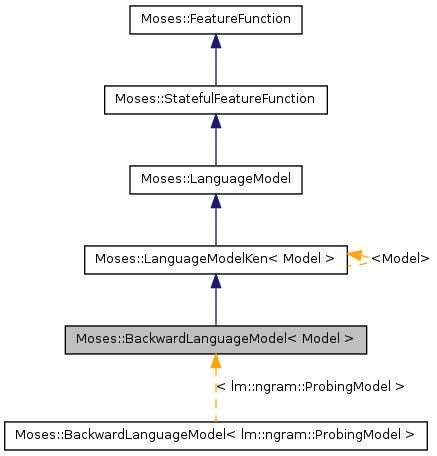[legend]
Collaboration diagram for Moses::BackwardLanguageModel< Model >: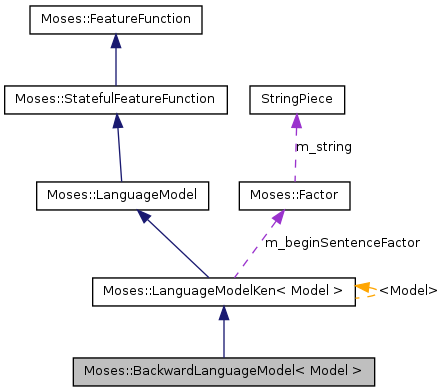[legend]

List of all members.

## Public Member Functions

BackwardLanguageModel (const std::string &line, const std::string &file, FactorType factorType, bool lazy)
virtual const FFStateEmptyHypothesisState (const InputType &) const
virtual void CalcScore (const Phrase &phrase, float &fullScore, float &ngramScore, size_t &oovCount) const
virtual FFStateEvaluate (const Hypothesis &hypo, const FFState *ps, ScoreComponentCollection *out) const
FFStateEvaluate (const Phrase &phrase, const FFState *ps, float &returnedScore) const

## Friends

class Moses::BackwardLanguageModelTest

## Detailed Description

### template<class Model> class Moses::BackwardLanguageModel< Model >

Definition at line 45 of file Backward.h.

## Constructor & Destructor Documentation

template<class Model >
 Moses::BackwardLanguageModel< Model >::BackwardLanguageModel ( const std::string & line, const std::string & file, FactorType factorType, bool lazy ) ` [inline]`

Constructs a new backward language model.

Definition at line 44 of file Backward.cpp.

## Member Function Documentation

template<class Model >
 void Moses::BackwardLanguageModel< Model >::CalcScore ( const Phrase & phrase, float & fullScore, float & ngramScore, size_t & oovCount ) const` [inline, virtual]`

Pre-calculate the n-gram probabilities for the words in the specified phrase.

Note that when this method is called, we do not have access to the context in which this phrase will eventually be applied.

In other words, we know what words are in this phrase, but we do not know what words will come before or after this phrase.

The parameters fullScore, ngramScore, and oovCount are all output parameters.

The value stored in oovCount is the number of words in the phrase that are not in the language model's vocabulary.

The sum of the ngram scores for all words in this phrase are stored in fullScore.

The value stored in ngramScore is similar, but only full-order ngram scores are included.

This is best shown by example:

Assume a trigram backward language model and a phrase "a b c d e f g"

fullScore would represent the sum of the logprob scores for the following values:

p(g) p(f | g) p(e | g f) p(d | f e) p(c | e d) p(b | d c) p(a | c b)

ngramScore would represent the sum of the logprob scores for the following values:

p(g) p(f | g) p(e | g f) p(d | f e) p(c | e d) p(b | d c) p(a | c b)

Reimplemented from Moses::LanguageModelKen< Model >.

Definition at line 123 of file Backward.cpp.

Referenced by Moses::BackwardLanguageModelTest::testCalcScore().

Here is the call graph for this function: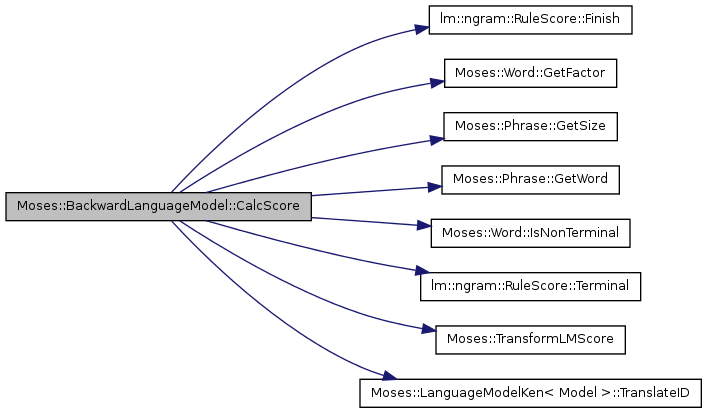Here is the caller graph for this function: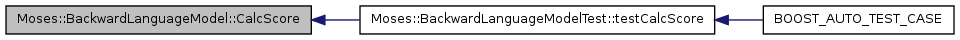template<class Model >
 const FFState * Moses::BackwardLanguageModel< Model >::EmptyHypothesisState ( const InputType & ) const` [inline, virtual]`

Constructs an empty backward language model state.

This state will correspond with a translation hypothesis where no source words have been translated.

In a forward language model, the language model state of an empty hypothesis would store the beginning of sentence marker <s>.

Because this is a backward language model, the language model state returned by this method instead stores the end of sentence marker </s>.

Reimplemented from Moses::LanguageModelKen< Model >.

Definition at line 63 of file Backward.cpp.

Here is the call graph for this function: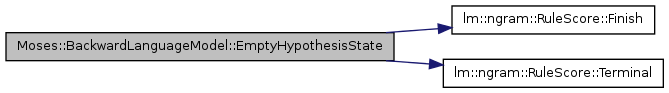Here is the caller graph for this function: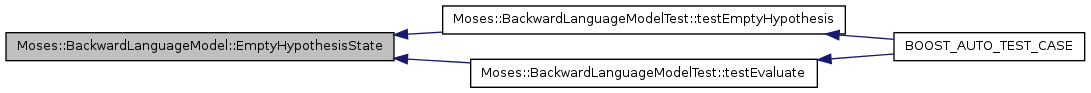template<class Model >
 FFState * Moses::BackwardLanguageModel< Model >::Evaluate ( const Phrase & phrase, const FFState * ps, float & returnedScore ) const` [inline]`

Definition at line 244 of file Backward.cpp.

Here is the call graph for this function: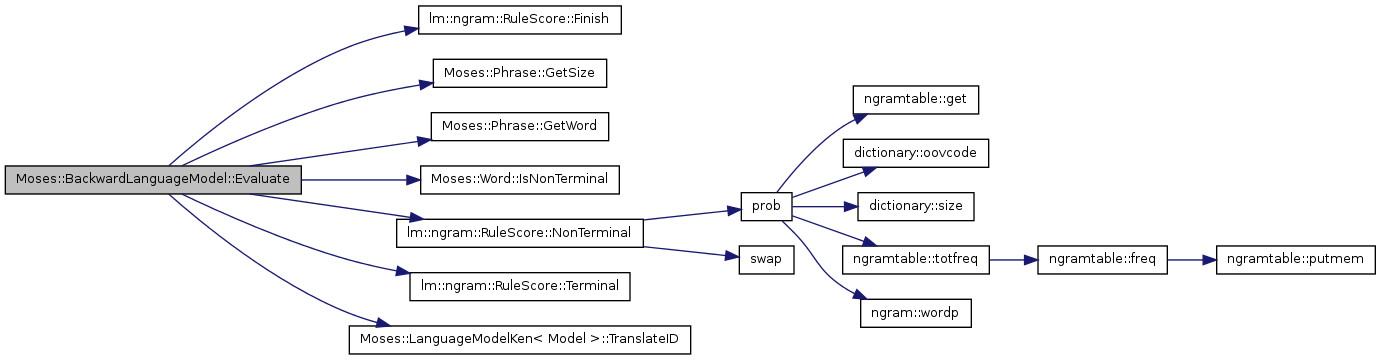template<class Model >
 FFState * Moses::BackwardLanguageModel< Model >::Evaluate ( const Hypothesis & hypo, const FFState * ps, ScoreComponentCollection * out ) const` [inline, virtual]`

Calculate the ngram probabilities for the words at the beginning (and under some circumstances, also at the end) of the phrase represented by the provided hypothesis.

Additionally, calculate a new language model state.

This is best shown by example:

Assume a trigram language model.

Assume the previous phrase was "a b c d e f g", which means the previous language model state is "g f".

When the phrase corresponding to "a b c d e f g" was previously processed by CalcScore the following full-order ngrams would have been calculated:

p(a | c b) p(b | d c) p(c | e d) p(d | f e) p(e | g f)

The following less-than-full-order ngrams would also have been calculated by CalcScore:

p(f | g) p(g)

In this method, we now have access to additional context which may allow us to compute the full-order ngrams for f and g.

Assume the new provided hypothesis contains the new phrase "h i j k"

Given these assumptions, this method is responsible for calculating the scores for the following:

p(f | h g) p(g | i h)

This method must also calculate and return a new language model state.

In this example, the returned language model state would be "k j"

If the provided hypothesis represents the end of a completed translation (all source words have been translated) then this method is additionally responsible for calculating the following:

p(j | <s> k) p(k | <s>)

Definition at line 219 of file Backward.cpp.

Referenced by Moses::BackwardLanguageModelTest::testEvaluate().

Here is the call graph for this function: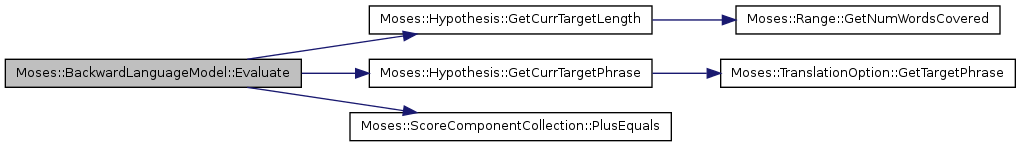Here is the caller graph for this function: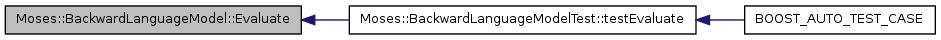## Friends And Related Function Documentation

template<class Model>
 friend class Moses::BackwardLanguageModelTest` [friend]`

Definition at line 67 of file Backward.h.

The documentation for this class was generated from the following files:
• /disk4/html/www/moses/doxygen/mosesdecoder/moses/LM/Backward.h
• /disk4/html/www/moses/doxygen/mosesdecoder/moses/LM/Backward.cpp

Generated on Thu Jul 6 00:33:19 2017 for Moses by1.5.9